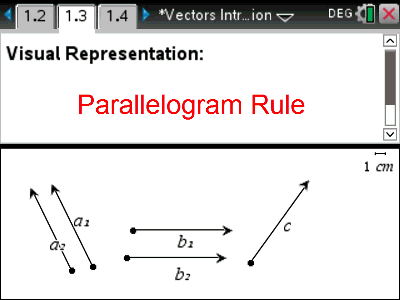# Activities

•30 Minutes

4.4

• ##### Report an Issue

Vectors Introduction#### Activity Overview

An introduction to vectors visually, conceptually and numerically. Students manipulate vectors to show addition (head to tail) and the parallelogram rule. Students use a column matrix to represent a vector and explore scalar multiples and the magnitude of a vector.

#### Objectives

• Add / Subtract vectors visually
• Add / Subtract vectors numerically
• Component form of a vector
• Magnitude of a vector
• Scalar product

• Scalar
• Vector
• Magnitude

#### About the Lesson

Students manipulate vectors adding them head to tail visually then progress to the matrix representation of a vector to see the numerical result. Students develop an understanding of the parallelogram rule to help understand that a + b = b + a. Dynamic objects allow students to see representations of a scalar multiplied by a vector and also the component form of a vector and how to calculate the magnitude of a vector with an informal introduction to the unit vector.